• 文件中包括代码，测试图片，以及代码使用的详细方法。
• 超像素分割为图像分割，图像处理的基础，文件为MATLAB和C混编的代码，demo为主程序，运行demo主程序进行超像素分割
• 超像素分割算法SLIC的matlab实现

千次阅读 多人点赞 2020-08-20 16:59:05
SLIC是一种基于网格化KMeans聚类的超像素分割算法，其计算复杂度为O(N)，其中N为像素点个数。SLIC的原理比较简单精致，具体的原理我这里就不介绍了，推荐大家自己去读原始论文加深理解。SLIC的算法流程如下： 如...

SLIC是一种基于网格化KMeans聚类的超像素分割算法，其计算复杂度为O(N)，其中N为像素点个数。SLIC的原理比较简单精致，具体的原理我这里就不介绍了，推荐大家自己去读原始论文加深理解（但我以为看下面这个算法流程图就足够理解原理了）。SLIC的算法流程如下：如所有其他聚类算法一样，SLIC不能保证连通性，所以需要后处理将旁生的连通域合并到邻近的主连通域上，但是论文中并未给出具体的后处理方法。我给出的方法是按照轮廓接触点个数最多原则合并连通域。由于每个聚类都有自己的“势力范围”，即每个标签覆盖的区域不会超过聚类时限定的范围（一般是2S*2S大小，边缘栅格的聚类例外），所以合并处理时只需要在该范围内操作即可。

下面给出本人实现的SLIC算法程序（控制色域与空域权重比例的系数wDs应设为函数形参，这里就不改了。注意！迭代中限定聚类的栅格是不变的！！！）：

function Label=SLIC(img,s,errTh,wDs)
% 基于KMeans的超像素分割
% img为输入图像，维度不限，最大值为255
% s x s为超像素尺寸
% errTh为控制迭代结束的联合向量残差上限
m=size(img,1);
n=size(img,2);

%% 计算栅格顶点与中心的坐标
h=floor(m/s);
w=floor(n/s);
rowR=floor((m-h*s)/2); %多余部分首尾均分
colR=floor((n-w*s)/2);
rowStart=(rowR+1):s:(m-s+1);
rowStart(1)=1;
rowEnd=rowStart+s;
rowEnd(1)=rowR+s;
rowEnd(end)=m;
colStart=(colR+1):s:(n-s+1);
colStart(1)=1;
colEnd=colStart+s;
colEnd(1)=colR+s;
colEnd(end)=n;
rowC=floor((rowStart+rowEnd-1)/2);
colC=floor((colStart+colEnd-1)/2);
% 显示划分结果
temp=zeros(m,n);
temp(rowStart,:)=1;
temp(:,colStart)=1;
for i=1:h
for j=1:w
temp(rowC(i),colC(j))=1;
end
end
figure,imshow(temp);
imwrite(temp,'栅格.bmp');

%% 计算梯度图像，使用sobel算子和欧式距离
img=double(img)/255;
r=img(:,:,1);
g=img(:,:,2);
b=img(:,:,3);
Y=0.299 * r + 0.587 * g + 0.114 * b;

f1=fspecial('sobel');
f2=f1';
gx=imfilter(Y,f1);
gy=imfilter(Y,f2);
G=sqrt(gx.^2+gy.^2);

%% 选择栅格中心点3*3邻域中梯度最小点作为起始点
rowC_std=repmat(rowC',[1,w]);
colC_std=repmat(colC,[h,1]);
rowC=rowC_std;
colC=colC_std;
for i=1:h
for j=1:w
block=G(rowC(i,j)-1:rowC(i,j)+1,colC(i,j)-1:colC(i,j)+1);
[minVal,idxArr]=min(block(:));
jOffset=floor((idxArr(1)+2)/3);
iOffset=idxArr(1)-3*(jOffset-1);
rowC(i,j)=rowC(i,j)+iOffset;
colC(i,j)=colC(i,j)+jOffset;
end
end

%% KMeans超像素分割
Label=zeros(m,n)-1;
dis=Inf*ones(m,n);
M=reshape(img,m*n,size(img,3)); %像素值重排
% 联合色域值和空域值
colorC=zeros(h,w,size(img,3));
for i=1:h
for j=1:w
colorC(i,j,:)=img(rowC(i),colC(j),:);
end
end
uniMat=cat(3,colorC,rowC,colC);
uniMat=reshape(uniMat,h*w,size(img,3)+2);
iter=1;
while(1)
uniMat_old=uniMat;
%     rowC_old=rowC;
%     colC_old=colC;
for k=1:h*w
c=floor((k-1)/h)+1;
r=k-h*(c-1);
rowCidx=rowC(r,c);
colCidx=colC(r,c); %聚类中心坐标
%聚类限定的栅格(中心点始终是原s x s栅格的中心点)
rowStart=max(1,rowC_std(r,c)-s);
rowEnd=min(m,rowC_std(r,c)+s-1);
colStart=max(1,colC_std(r,c)-s);
colEnd=min(n,colC_std(r,c)+s);
%         colorC=uniMat(k,1:size(img,3));
colorC=M((colCidx-1)*m+rowCidx,:);
for i=rowStart:rowEnd
for j=colStart:colEnd
colorCur=M((j-1)*m+i,:);
dc=norm(colorC-colorCur);
ds=norm([i-rowCidx,j-colCidx]);
d=dc^2+wDs*(ds/s)^2;
if d<dis(i,j)
dis(i,j)=d;
Label(i,j)=k;
end
end
end
end

%显示聚类结果
temp=mod(Label,20)+1;
figure;
imagesc(label2rgb(temp-1,'jet','w','shuffle')) ;
axis image ; axis off ;
% 录制gif
F=getframe(gcf);
I=frame2im(F);
[I,map]=rgb2ind(I,256);
if iter == 1
imwrite(I,map,'test.gif','gif','Loopcount',inf,'DelayTime',0.2);
else
imwrite(I,map,'test.gif','gif','WriteMode','append','DelayTime',0.2);
end
iter=iter+1;

% 更新聚类中心
colorC=zeros(h,w,size(img,3));
for k=1:h*w
num=0;
sumColor=zeros(1,size(img,3));
sumR=0;
sumC=0;
c=floor((k-1)/h)+1;
r=k-h*(c-1);
rowCidx=rowC_std(r,c);
colCidx=colC_std(r,c);
rowStart=max(1,rowCidx-s);
rowEnd=min(m,rowCidx+s-1);
colStart=max(1,colCidx-s);
colEnd=min(n,colCidx+s);

for row=rowStart:rowEnd
for col=colStart:colEnd
if Label(row,col)==k
num=num+1;
sumR=sumR+row;
sumC=sumC+col;
color=reshape(img(row,col,:),1,size(img,3));
sumColor=sumColor+color;
end
end
end
colorC(r,c,:)=sumColor/num;
rowC(r,c)=round(sumR/num);
colC(r,c)=round(sumC/num);
end
uniMat=cat(3,colorC,rowC,colC);
uniMat=reshape(uniMat,h*w,size(img,3)+2);
diff=uniMat-uniMat_old;
diff(:,1:2)=sqrt(wDs)*diff(:,1:2)/s;
err=norm(diff)/sqrt(h*w);
if err<errTh %残差低于阈值，结束迭代
break;
end
end

%% 后处理， 按照边界接触点数最多原则分配小连通域的标签
for k=1:h*w
c=floor((k-1)/h)+1;
r=k-h*(c-1);
rowCidx=rowC_std(r,c);
colCidx=colC_std(r,c);
rowStart=max(1,rowCidx-s);
rowEnd=min(m,rowCidx+s-1);
colStart=max(1,colCidx-s);
colEnd=min(n,colCidx+s);
block=Label(rowStart:rowEnd,colStart:colEnd);
block(block~=k)=0;
block(block==k)=1;
label=bwlabel(block);
szlabel=max(label(:)); %标签个数
bh=rowEnd-rowStart+1;
bw=colEnd-colStart+1;  %block的宽高

if szlabel<2  %无伴生连通域，略过
continue;
end

labelC=label(rowCidx-rowStart+1,colCidx-colStart+1); %主连通域的标记值
top=max(1,rowStart-1);
bottom=min(m,rowEnd+1);
left=max(1,colStart-1);
right=min(n,colEnd+1);
for i=1:szlabel %遍历连通域
if i==labelC %主连通域不处理
continue;
end
marker=zeros(bottom-top+1,right-left+1); %生成一个外扩一圈的marker，标记哪些点已经被统计过接触情况
bw=label;
bw(bw~=i)=0;
bw(bw==i)=1; %当前连通域标记图
contourBW=bwperim(bw); %求取外轮廓
%             figure,imshow(contourBW);
idxArr=find(double(contourBW)==1);
labelArr=zeros(4*length(idxArr),1);  %记录轮廓点的4邻域点标记值的向量
num=0;
for idx=1:size(idxArr) %遍历轮廓点,统计其4邻域点的标记值
bc=floor((idxArr(idx)-1)/bh)+1;
br=idxArr(idx)-bh*(bc-1); %轮廓点在block中的行列信息
row=br+rowStart-1;
col=bc+colStart-1; %轮廓点在大图中的行列信息
rc=[row-1,col;...
row+1,col;...
row,col-1;...
row,col+1];
for p=1:4
row=rc(p,1);
col=rc(p,2);

if ~(row>=1 && row<=m && col>=1 && col<=n && Label(row,col)~=k)
continue;
end

if marker(row-top+1,col-left+1)==0 %未被统计过
marker(row-top+1,col-left+1)=1;
num=num+1;
labelArr(num)=Label(row,col);
end
end
end

labelArr(find(labelArr==0))=[]; %去除零元素
uniqueLabel=unique(labelArr);
numArr=zeros(length(uniqueLabel),1);
for p=1:length(uniqueLabel)
idx=find(labelArr==uniqueLabel(p));
numArr(p)=length(idx);
end
idx=find(numArr==max(numArr));
maxnumLabel=uniqueLabel(idx(1)); %接触最多的标签

for row=rowStart:rowEnd
for col=colStart:colEnd
if bw(row-rowStart+1,col-colStart+1)==0
continue;
end
Label(row,col)=maxnumLabel;
end
end
end
end

% 显示连通域处理后聚类结果
temp=mod(Label,20)+1;
figure;
imagesc(label2rgb(temp-1,'jet','w','shuffle')) ;
axis image ; axis off ;

脚本文件：

close all;clc;
figure,imshow(I);

s=15;
errTh=10^-2;
wDs=0.5^2;
Label=SLIC(I,s,errTh,wDs);

%% 显示轮廓
marker=zeros(size(Label));
[m,n]=size(Label);
for i=1:m
for j=1:n
top=Label(max(1,i-1),j);
bottom=Label(min(m,i+1),j);
left=Label(i,max(1,j-1));
right=Label(i,min(n,j+1));
if ~(top==bottom && bottom==left && left==right)
marker(i,j)=1;
end
end
end
figure,imshow(marker);

I2=I;
for i=1:m
for j=1:n
if marker(i,j)==1
I2(i,j,:)=0;
end
end
end
figure,imshow(I2);

测试图像：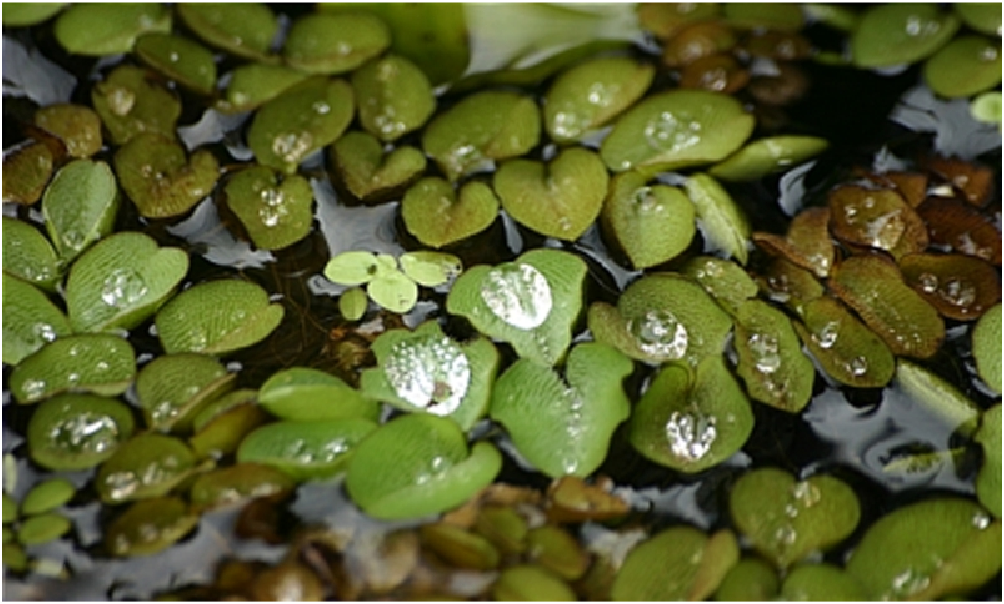栅格划分结果：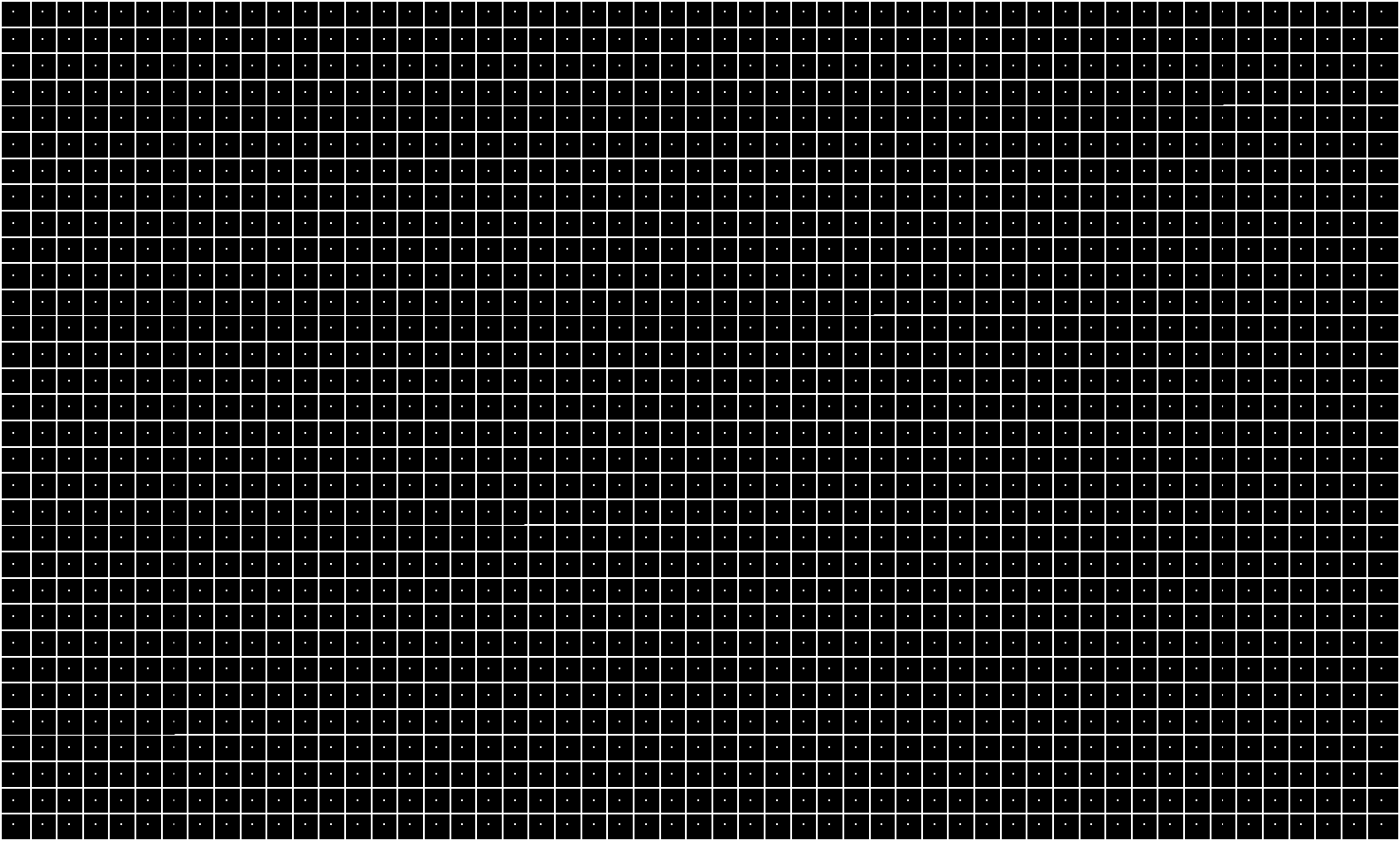聚类过程：聚类最终结果：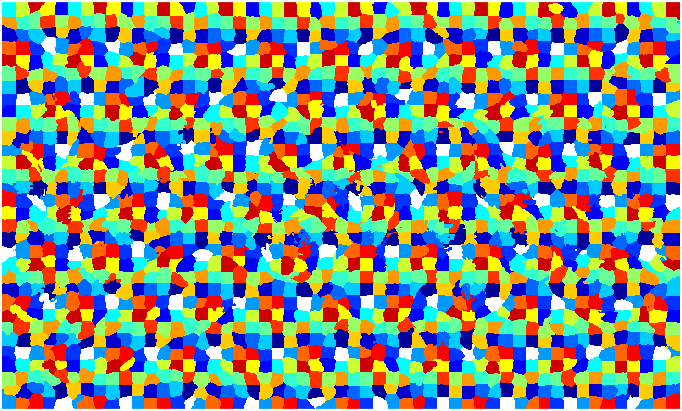连通域合并后的结果：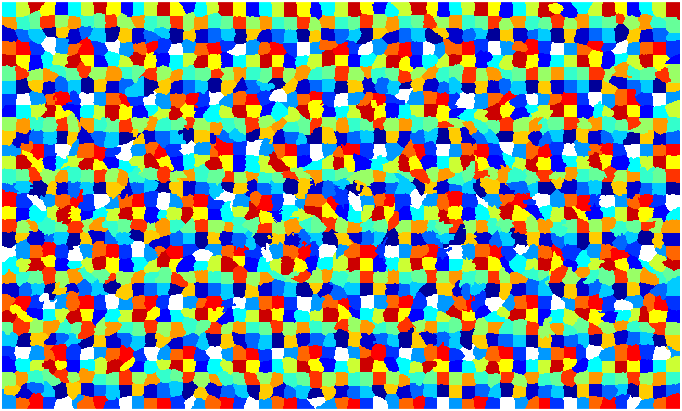原图+轮廓线：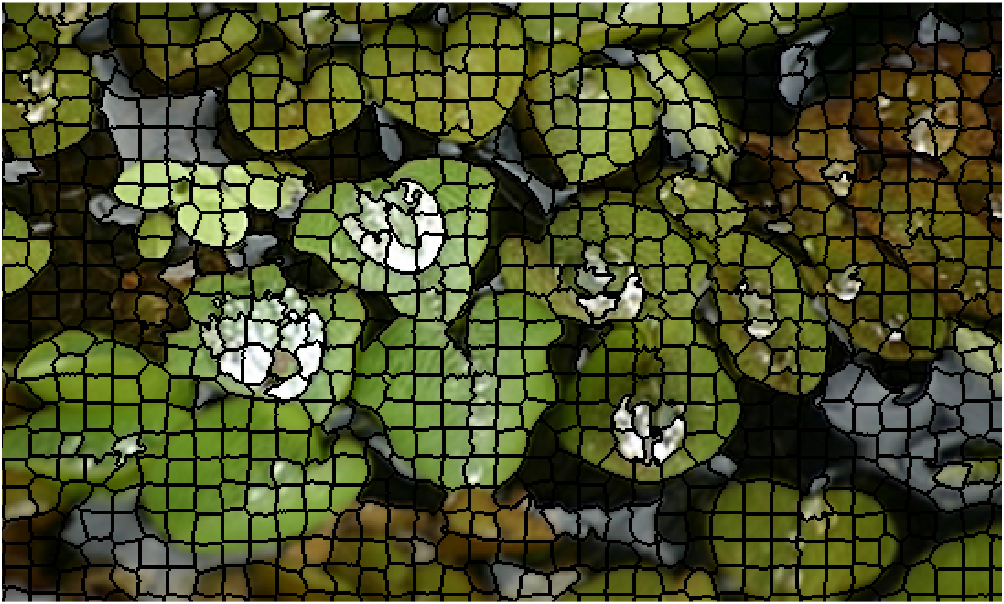具体的我就不解释了，自觉程序写得还是很有条理的，读者自己跟踪程序运行进行理解吧。

展开全文• 超像素分割代码 超像素概念是2003年Xiaofeng Ren提出和发展起来的图像分割技术，是指具有相似纹理、颜色、亮度等特征的相邻像素构成的有一定视觉意义的不规则像素块。它利用像素之间特征的相似性将像素分组,用少量的...
• SLIC超像素分割算法MATLAB算法代码实现，超像素分割关键代码形式
• SLIC的matlab代码，可以更改自己的图片位置，生成结果，可以直接运行使用
• 本代码是论文: Liu M Y, Tuzel O, Ramalingam S, et al. Entropy rate superpixel segmentation[C]// Computer Vision and Pattern Recognition. IEEE, 2011:2097-2104. 的实现代码，网络上的原始链接均已失效，分享...
• 本文介绍了一种基于图论的超像素分割及其合并算法,主要在检测图像的边界,图的分割准则,超像素区域特征的提取,度量超像素区域间的相似性这几个方面进行了研究. 首先,针对图像的边界检测这一问题,本文对最...

摘要：

超像素分割是计算机视觉中的热点问题,超像素的使用能有效地减少图像局部信息的冗余,大大降低了图像处理的复杂度和运算量,而且还保留了对图像进行进一步处理的有效信息,超像素技术越来越广泛地应用于许多领域.本文介绍了一种基于图论的超像素分割及其合并算法,主要在检测图像的边界,图的分割准则,超像素区域特征的提取,度量超像素区域间的相似性这几个方面进行了研究. 首先,针对图像的边界检测这一问题,本文对最近比较好的图像边界检测方法进行简单的介绍,通过实验对比和分析选取最优的边界检测方法,其边界检测结果作为本文超像素分割算法的输入.以图论为基础,介绍一种超像素分割准则,最后研究了一种把边界检测方法和图论算法相结合的超像素分割算法,此超像素分割算法使基于图论的分割方法能够分割像素分辨率较高的图像,改变了以往基于图论的超像素分割算法只能分割分辨率较低的图像的现状. 然后,在相似性测量方面,利用图像的低水平信息,例如颜色特征,纹理特征,位置特征,形状特征,设计了一种新的度量两个超像素区域之间相似性的方法.图像经过超像素分割算法分割得到超像素区域后,根据相似性度量方法测量每个超像素区域与其相邻超像素区域之间的相似性. 最后,针对图像分割中的过分割现象,本文利用研究的的超像素区域间相似性度量方法,计算超像素间的相似性,然后根据选定的阈值判断是否把它们进行合并,超像素合并算法可以使超像素分割算法中存在的过分割现象得到很好的改善,并且打破了以前仅仅运用图像的颜色和位置这些信息进行区域合并的限制.

展开

展开全文• 直观效果上看，DBSCAN算法可以找到样本点的全部密集区域，并把这些密集区域当做一个一个的聚类簇。 2个算法参数：邻域半径R和最少点数目minpoints 这两个算法参数实际可以刻画什么叫密集——当邻域半径R内的点的...

一、获取代码方式

获取代码方式1：
通过订阅紫极神光博客付费专栏，凭支付凭证，私信博主，可获得此代码。

获取代码方式2：
通过紫极神光博客主页开通CSDN会员，凭支付凭证，私信博主，可获得此代码。

获取代码方式3：
完整代码已上传我的资源：【图像分割】基于matlab DBSCAN算法超像素分割【含Matlab源码 515期】

备注：开通CSDN会员，仅只能免费获得1份代码（有效期为开通日起，三天内有效）；
订阅紫极神光博客付费专栏，可免费获得1份代码（有效期为订阅日起，三天内有效）；

三、部分源代码

%======================================================================
clear all;
close all;
currentFolder = pwd;
name='107072';
post=1;
img = uint8(im);
number_superpixels =500;
tic;
label = DBscan_mex(img,number_superpixels,post);
toc;
SuperpixelSave(label,im,name);
DisplaySuperpixel(label,im,name);
image=rgb2gray(img);
G=imbinarize(image);
B = imresize(G, 0.125);
%% 这是Roberts算子
J = imresize(sourcePic, 0.125);
grayPic=mat2gray(J); %转换成灰度图像
[m,n]=size(grayPic);
newGrayPic=grayPic;%为保留图像的边缘一个像素
robertsNum=0; %经roberts算子计算得到的每个像素的值
robertThreshold=0.2; %设定阈值
for j=1:m-1 %进行边界提取
for k=1:n-1
robertsNum = abs(grayPic(j,k)-grayPic(j+1,k+1)) + abs(grayPic(j+1,k)-grayPic(j,k+1));
if(robertsNum > robertThreshold)
newGrayPic(j,k)=255;
else
newGrayPic(j,k)=0;
end
end
end
A=imbinarize(newGrayPic);
sum1=0;
sum2=0;
[m,n]=size(A);%lb=length(Y);
%[r,k]=size(B);
for i=1:n
%for q=1:k
for j=1:m
%for p=1:r
while A(i,j)==1
sum2=sum2+1;
if A(i,j)-B(i,j)<2 || A(i,j)-B(i-1,j-1)<2 || A(i,j)-B(i,j-1)<2||...
A(i,j)-B(i-1,j+1)<2|| A(i,j)-B(i+1,j)<2 || A(i,j)-B(i+1,j+1)<2 ||...
A(i,j)-B(i+1,j)<2||A(i,j)-B(i-1,j+1)<2 ||A(i,j)-B(i-1,j)<2
sum1=sum1+1;
end
function DisplaySuperpixel(label,img,name)

[nRows,nCols,~]=size(img);
for m=1:nRows
for n=1:nCols
L=label(m,n);
count=0;
minx=max(m-1,1);
maxx=min(m+1,nRows);
miny=max(n-1,1);
maxy=min(n+1,nCols);
for u=minx:maxx;
for v=miny:maxy
if(label(u,v)~=L)
count=count+1;
end
if(count==2)
break;
end
end
if(count==2)
break;
end
end
if(count==2)
img(m,n,:)=0;
end
end
end
figure;
imshow(img);
imwrite(img,[name,'result.bmp'],'bmp')

fid=fopen([name,'.txt'],'wt');%写入文件路径
[m,n]=size(label);
for i=1:1:m
for j=1:1:n
if j==n
fprintf(fid,'%g\n',label(i,j));
else
fprintf(fid,'%g\t',label(i,j));
end
end

四、运行结果五、matlab版本及参考文献

1 matlab版本
2014a

2 参考文献
 蔡利梅.MATLAB图像处理——理论、算法与实例分析[M].清华大学出版社，2020.
杨丹,赵海滨,龙哲.MATLAB图像处理实例详解[M].清华大学出版社，2013.
周品.MATLAB图像处理与图形用户界面设计[M].清华大学出版社，2013.
刘成龙.精通MATLAB图像处理[M].清华大学出版社，2015.
赵勇,方宗德,庞辉,王侃伟.基于量子粒子群优化算法的最小交叉熵多阈值图像分割[J].计算机应用研究. 2008,(04)

展开全文matlab 算法
• 基于超像素的图像分割；使用matlab编程，运行main.m文件。
• 代码网址：http://ivrl.epfl.ch/research/superpixels#SLICO PS：上面的链接失效了，我搞了个代码托管，附链接：... 里面的“SLIC_mex.zip”应该是作者官网上下载的。 下载了SLIC超像素分割的MATL...

更新：评论区有同学分享了新的代码链接，贴在这里吧：

https://www.epfl.ch/labs/ivrl/research/slic-superpixels/

***************************************************   分       割        线    ******************************************************

代码网址：http://ivrl.epfl.ch/research/superpixels#SLICO

里面的“SLIC_mex.zip”应该是作者官网上下载的。

下载了SLIC超像素分割的MATLAB程序：

下载解压之后，主程序是“SLICdemo.m”，它会调用C语言程序“slicmex.c”。

所以运行程序的时候，首先通过MATLAB将C/C++函数编译成MEX函数，在MATLAB中就可以调用了。方法是：

MATLAB的command window下输入：

>>mex silcmex.c

会通知你选择编译器：

Select a compiler:
 Microsoft Visual C++ 2010 in d:\ruanjiananzhuang\VS2010UltimTrialCHS

 None

Compiler: 1

输入1，回车，这时就会报出警告和错误，警告不用管，主要解决错误：

slicmex.c(387) : error C2275: “mwSize”: 将此类型用作表达式非法

......

这个错误是最先出现的最大的错误，先解决它。

用文本编辑器打开文件“slicmex.c”，处理（387）行：

mwSize numdims = mxGetNumberOfDimensions(prhs) ;

改法就是：将numdims的声明和定义拆开：

mwSize numdims;

numdims = mxGetNumberOfDimensions(prhs) ;

再将其声明与（351）行定义的mexFunction函数下面的一大串变量声明放在一起。截图如下：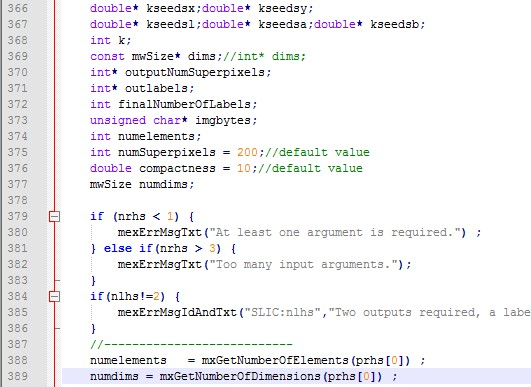保存文件，再返回到MATLAB的命令窗口处，重新编译c程序：

>> mex slicmex.c

再运行程序：
>> SLICdemo

网上有人给出了原理，我把文字复制过来：

error C2275: “XXX”: 将此类型用作表达式非法

在移植c++代码到c的时候，经常会出现一个奇怪的错误，
error C2275: “XXX”: 将此类型用作表达式非法
表达式非法，这个错误是由于c的编译器要求将变量的申明放在一个函数块的头部，而c++没有这样的要求造成的。
解决的办法就是把变量的申明全部放在变量的生存块的开始。

展开全文SLIC超像素分割
• 简介：最近项目使用到了超像素分割，因此顺道研究了以下SLIC这一算法超像素分割这类low-level vision问题已经在CVPR，ICCV这种顶级会议上逐渐销声匿迹，越来越流行的learning method渐渐占据了这些顶级会议90%的...超像素 图像处理 深度学习 python
• 超像素分割算法，亲测可用，内含数据图片，不用mex，直接matlab运行
• 写完这篇，图像分割的传统方法就快全了，传统图像分割大体有基于阈值的，这类就没啥算法可以写，所以直接略过了；...最后就是超像素分割了，超像素分割有k-means算法的影子，所以可以先看看k-means算法的代码实现过程.算法 聚类 opencv 图像处理 超像素分割
• SLIC超像素分割算法

万次阅读 多人点赞 2017-09-22 18:17:13
超像素概念是2003年Xiaofeng Ren提出和发展起来的图像分割技术，是指具有相似纹理、颜色、亮度等特征的相邻像素构成的有一定视觉意义的不规则像素块。它利用像素之间特征的相似性将像素分组...几种常见的超像素分割方法超像素分割算法
• 原图与分割结果 ...%用Superpixels算法对图像进行分割 I=imread('D:\Gray Files\lena.jpg'); %提取各颜色分量 B = double(I(:,:,3)); G = double(I(:,:,2)); R = double(I(:,:,1)); %种子点数量 nu...图像分割 超像素
• SLIC超像素分割MATLAB代码SLIC 超像素 该存储库提供了带有 Python 和 Matlab 接口的简单线性迭代聚类 (SLIC) 算法的代码。 在这两种情况下，都提供了一个演示文件，应该很容易使用。 这两个版本都可以为灰色、彩色...
• SLIC： simple linear ... 这是一个基于聚类算法超像素分割，由LAB空间以及x、y像素坐标共5维空间来计算。不仅可以分割彩色图，也可以兼容分割灰度图，它还有一个优点就是可以人为的设置需要分割的超像素的数量。
• 简单，可运行，代码只有几行，直接调用函数，图片都有。
• 不提供针对算法的学习，仅提供在matlab环境下的调用以完成SLIC超像素分割的功能。 调用格式如下： I = imread('你要读的图像路径'); [height,width,ch]=size(I); J = zeros(height,width,'uint32'); K = uint32(I); ...
• 基于超像素的图像分割,基于超像素的图像分割算法,matlab源码
• 源代码为C代码、需编译成MATLAB可执行文件后使用。经本人使用验证有效。。有问题的可以私信。。该代码确定可以实现相应的功能。。SLIC
• 预期分割matlab代码超级像素生成 该程序为P. Felzenszwalb的基于图的图像分割代码提供了MATLAB包装器。 有关原始C ++实现的信息，请参考。 有关算法的详细信息，请参阅论文“ Graph Based Image Segmentation 。 ...
• 超像素分割方法，采用论文 SEEDS: Superpixels Extracted via Energy-Driven Sampling 提出的方法，对于物体的边界具有较好的保留，如下图所示。可以辅助目标检测中制作Banchmark。 本工程在其基础上，获得的图像...
• 介绍SLIC超像素分割算法，给出其与openCV的接口，代码用VS2012和openCV2.4.9测试可运行。opencv
• 线性光谱聚类（LSC）的超像素分割算法，该算法可以生成具有低计算成本的紧凑且均匀的超像素。基本上，基于测量图像像素之间的颜色相似性和空间接近度的相似性度量，采用超像素分割的归一化切割公式。然而，代替使用...matlab
• 题目如下： 简单解法（HSV 直方图阈值）如下： ...这一节先讲SLIC超像素算法，下一节讲在超像素基础上用Kmeans分类进行分割，参考博客如下： https://www.jianshu.com/p/d0ef931b3ddf https://blog.csdn.net/duyue...opencv c++ SLIC 超像素...

超像素分割算法matlabmatlab 订阅